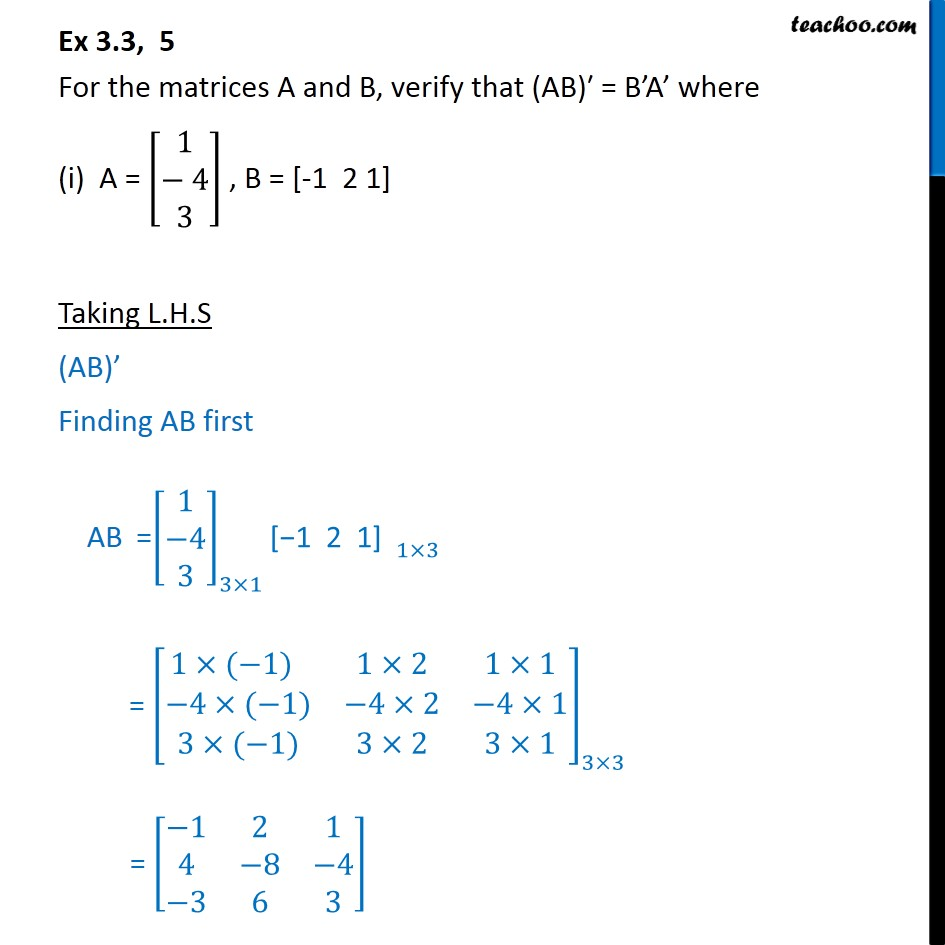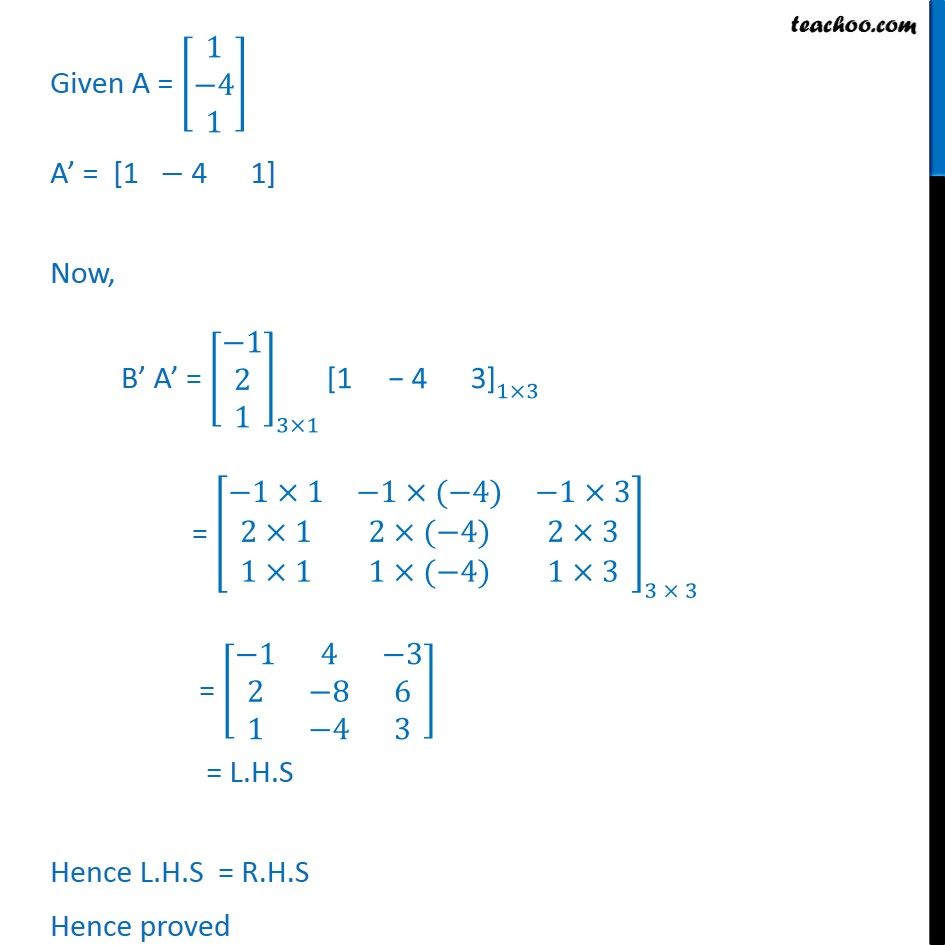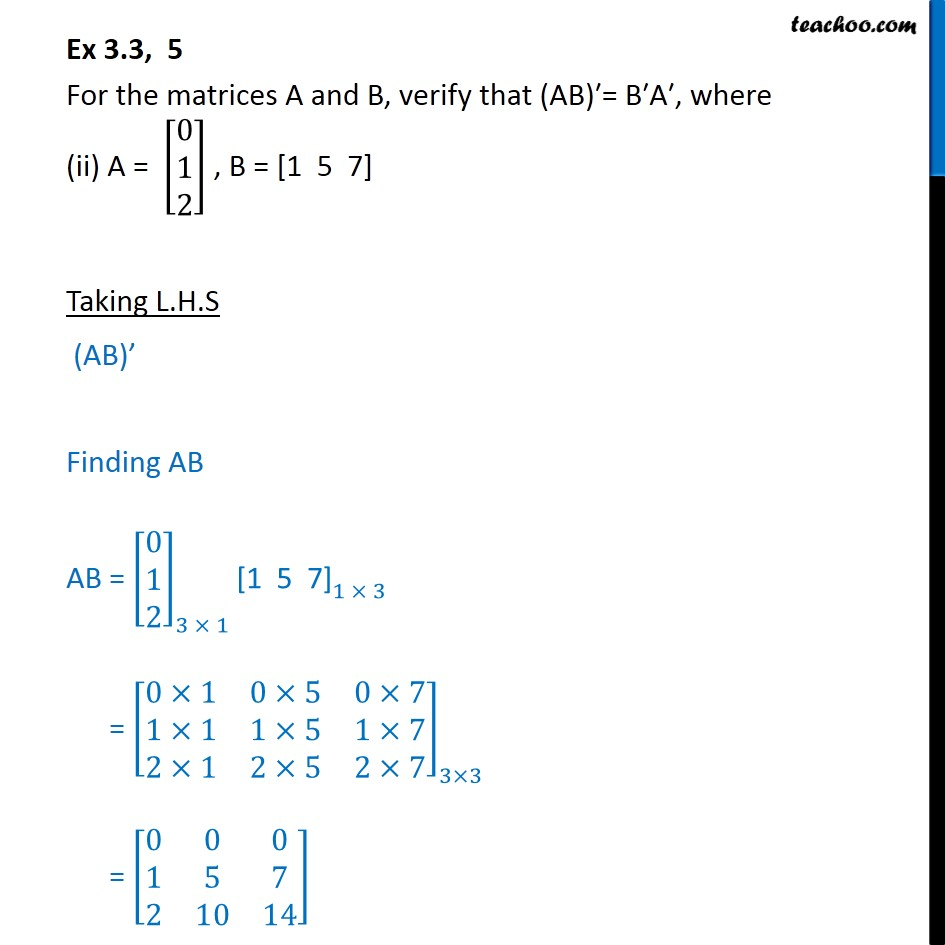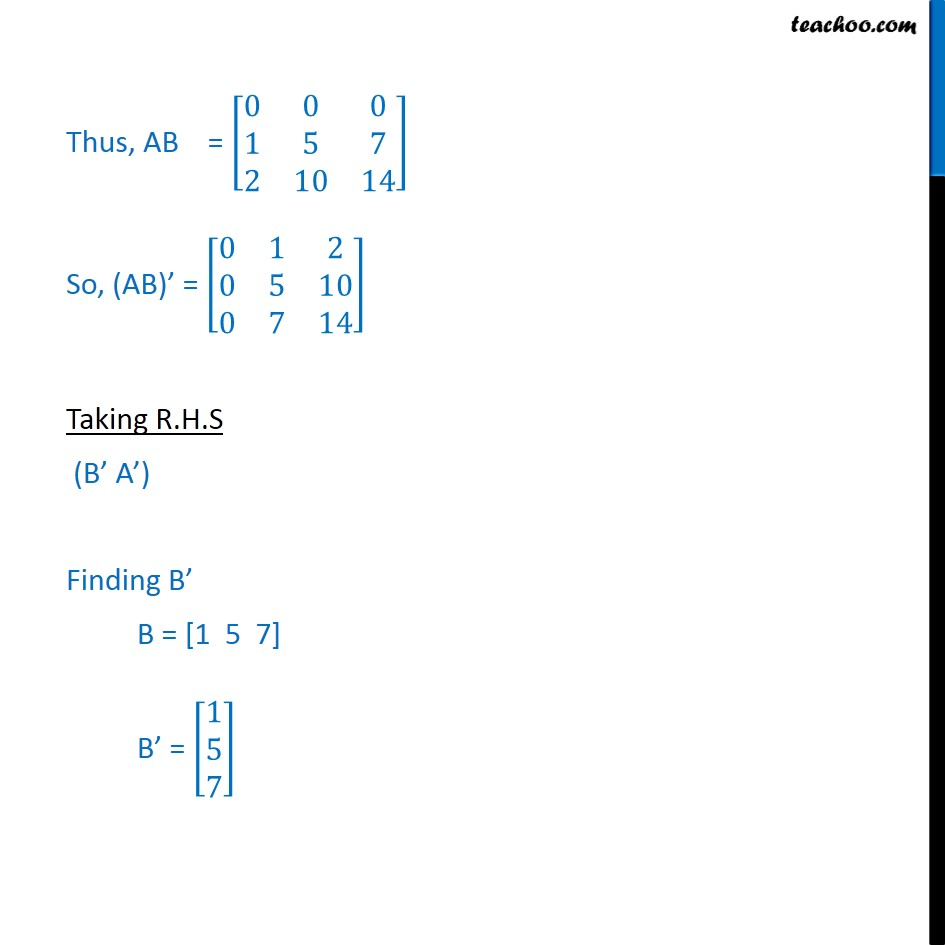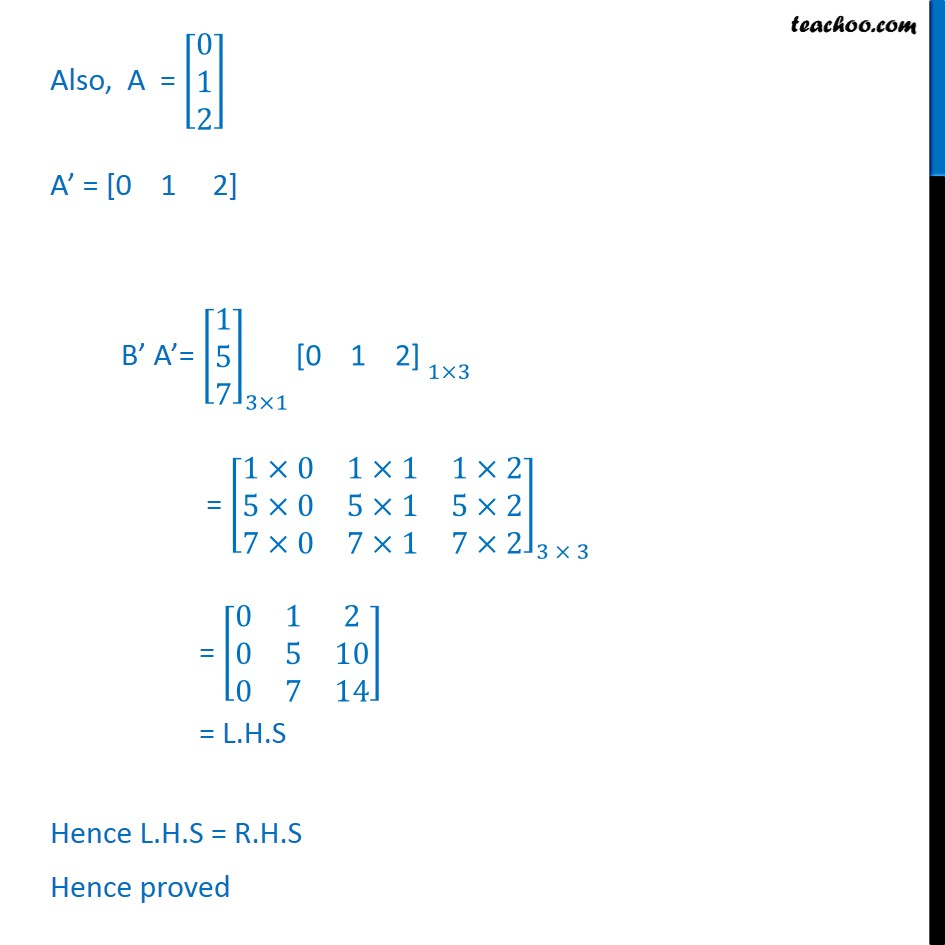1. Chapter 3 Class 12 Matrices
2. Serial order wise
3. Ex 3.3

Transcript

Ex 3.3, 5 For the matrices A and B, verify that (AB) = B A where (i) A = [ 8(1@ 4@3)] , B = [-1 2 1] Taking L.H.S (AB) Finding AB first AB =[ 8(1@ 4@3)]_(3 1) "[ 1 2 1] " _(1 3) = [ 8(1 ( 1) &1 2&1 1@ 4 ( 1)& 4 2& 4 1@3 ( 1)&3 2&3 1)]_(3 3) = [ 8( 1&2&1@4& 8& 4@ 3&6&3)] AB = [ 8( 1&2&1@4& 8& 4@ 3&6&3)] Now, (AB) = [ 8( 1&4& 3@2& 8&6@1& 4&3)] Taking R.H.S B A Finding B , A Given B = [ 1 2 1] B = "[" "1 2 1]" ^ = [ 8( 1@2@1)] Given A = [ 8(1@ 4@1)] A = [1 4 1] Now, B A = [ 8( 1@2@1)]_(3 1) "[1 4 3]" _(1 3) = [ 8( 1 1& 1 ( 4)& 1 3@2 1&2 ( 4)&2 3@1 1&1 ( 4)&1 3)]_(3 3) = [ 8( 1&4& 3@2& 8&6@1& 4&3)] = L.H.S Hence L.H.S = R.H.S Hence proved Ex 3.3, 5 For the matrices A and B, verify that (AB) = B A , where (ii) A = [ 8(0@1@2)] , B = [1 5 7] Taking L.H.S (AB) Finding AB AB = [ 8(0@1@2)]_(3 1) "[1 5 7]" _(1 3) = [ 8(0 1&0 5&0 7@1 1&1 5&1 7@2 1&2 5&2 7)]_(3 3) = [ 8(0&0&0@1&5&7@2&10&14)] Thus, AB = [ 8(0&0&0@1&5&7@2&10&14)] So, (AB) = [ 8(0&1&2@0&5&10@0&7&14)] Taking R.H.S (B A ) Finding B B = [1 5 7] B = [ 8(1@5@7)] Also, A = [ 8(0@1@2)] A = [0 1 2] B A = [ 8(1@5@7)]_(3 1) "[0 1 2] " _(1 3) = [ 8(1 0&1 1&1 2@5 0&5 1&5 2@7 0&7 1&7 2)]_(3 3) = [ 8(0&1&2@0&5&10@0&7&14)] = L.H.S Hence L.H.S = R.H.S Hence proved

Ex 3.3

Chapter 3 Class 12 Matrices
Serial order wise